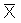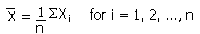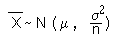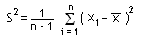## Random Samples

A random sample is a collection of independent random variables X1, X2,..., Xn, all with the same probability distribution.

The Sample Mean

The sample mean,of a random sample X1, ... Xn is given by:If the random varables each have a normal distribution (with mean m and variance s2), then the sample mean has a normal distribution with mean m and variance s2/n, in other words:Expectation and Varianceis itself a random variable and so has an expectation and variance.

These are easy to calculate, for example:

E() = E ( SXi/n ) = (1/n)E( X1 + X2 + ... + Xn) = (1/n)[E(X1) + E(X2) + ... + E(Xn)]

If each of the random variables X1, ... Xn have expectation m, then this is equal to (1/n)(n m) = m .

• E() = m

Similarly, if each of the random variables X1, ... Xn has variance s2, it can be shown that:

• Var() = (s2)/n

The Sample Variance

The sample variance is given by: